# SSAT Upper Level Math : How to read graphs

## Example Questions

### Example Question #1 : How To Read Graphs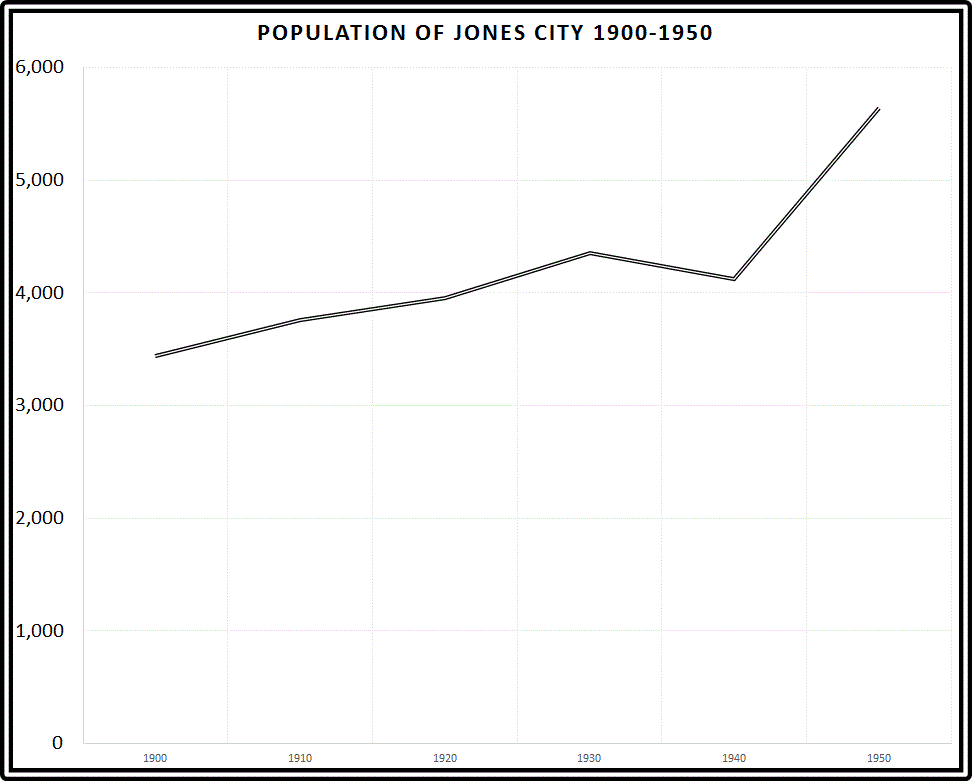Refer to the above graph. Which of the following comes closest to the percent of increase in population enjoyed by Jones City between 1900 and 1950?

20% increase

40% increase

100% increase

80% increase

60% increase

60% increase

Explanation:

In 1900, the population was about 3,500; in 1950, it was about 5,600. A reasonable estimate of the percent of increase would be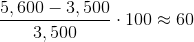so 60% would be the most reasonable choice.

### Example Question #2 : How To Read Graphs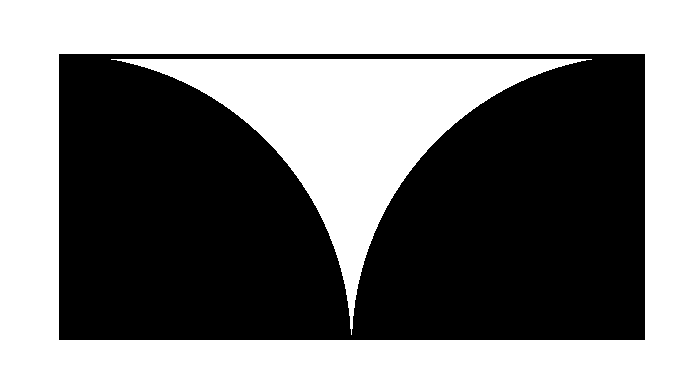The above figure shows two black quarter-circles inscribed inside a rectangle. If the black region has area 200, what is the area of the white region (nearest tenth)?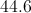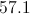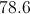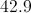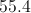Explanation:

If the radius of each quarter-circle is, the black region has area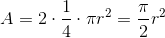Set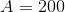and solve for: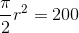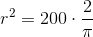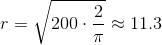The area of the rectangle, which we will call, is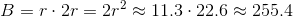The area of the white region is the difference of these two areas, or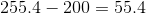### Example Question #3 : How To Read Graphs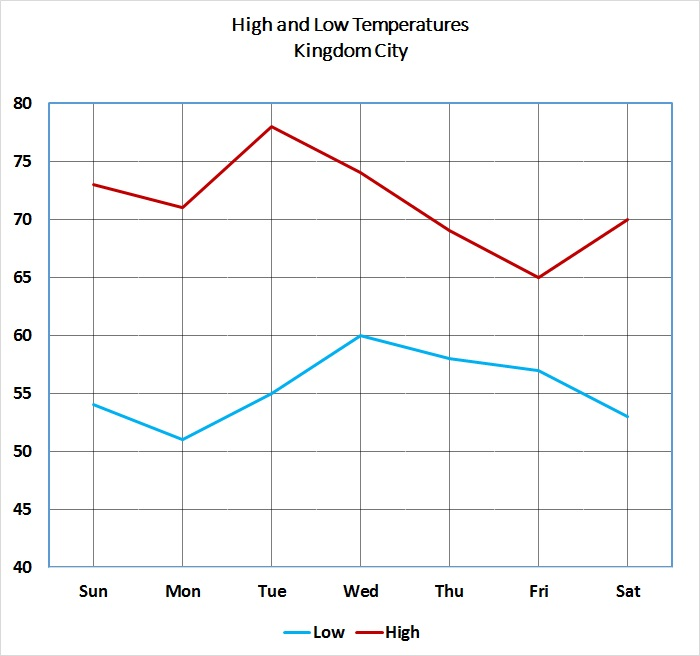Refer to the above graph, which shows the high and low temperatures for Kingdom City over a one-week period.

Tim wants to know the high temperature for the Sunday of the next week. He does not have access to that information, but he knows that it was higher than those of exactly four of the five previous days, and lower than that of one of them. Which of the following temperatures could have been the high temperature for that day?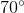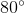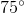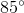Explanation:

Since we are dealing with the day after the last one shown, and we are comparing that day's high temperature to those of the previous five days, we only need to concern ourselves with the high temperatures for Tuesday through Saturday.

Examine the figure below, which shows the high temperature line for those five days.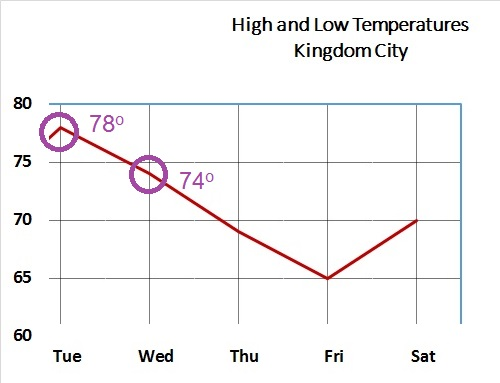For the high temperature for the next Sunday to be greater than exactly four of the five and less than the remaining one, the temperature must be strictly between the highest and second-highest high temperatures, which are seen to be 78 and 74 degrees, respectively. That is, if we letbe the temperature in degrees,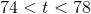Of the four choices, only 75 falls in this range.

### Example Question #4 : How To Read GraphsRefer to the above graph, which shows the high and low temperatures for Kingdom City over a one-week period.

Which of the following temperatures occurred at least once on each of the seven days?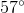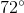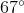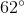Explanation:

The graph is recreated in the figure below, with horizontal lines at the 57, 62, 67, and 72 degree levels.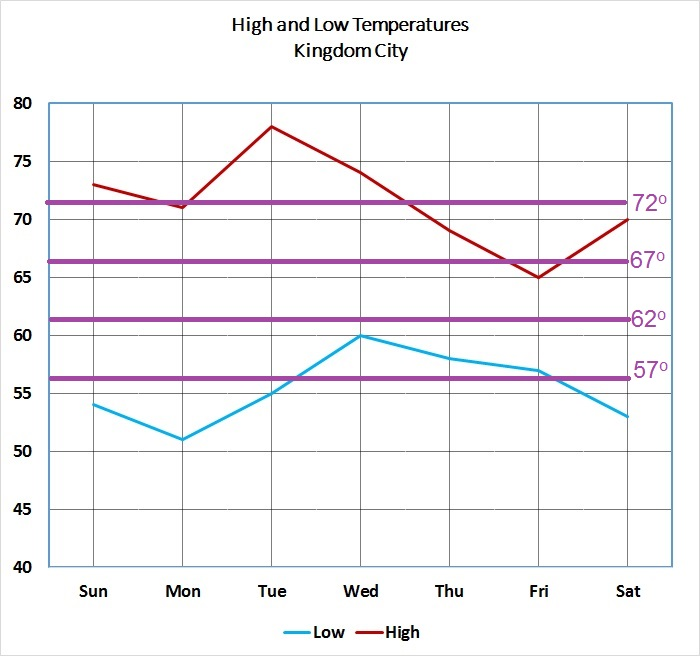It can be seen that of the four lines, only the 62 degree line falls between the high temperature and low temperature for each of the seven days. This is the correct choice.

### Example Question #5 : How To Read GraphsRefer to the above graph, which shows the high and low temperatures for Kingdom City over a one-week period. The temperatures at left are given in degrees Fahrenheit.

Temperatures given in degrees Fahrenheit can be converted to the Celsius scale using the formula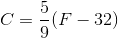.

Express the lowest temperature of the week in degrees Celsius (nearest whole degree).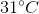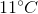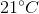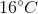Explanation:

As can be seen below, the lowest temperature for the week was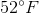, on Monday.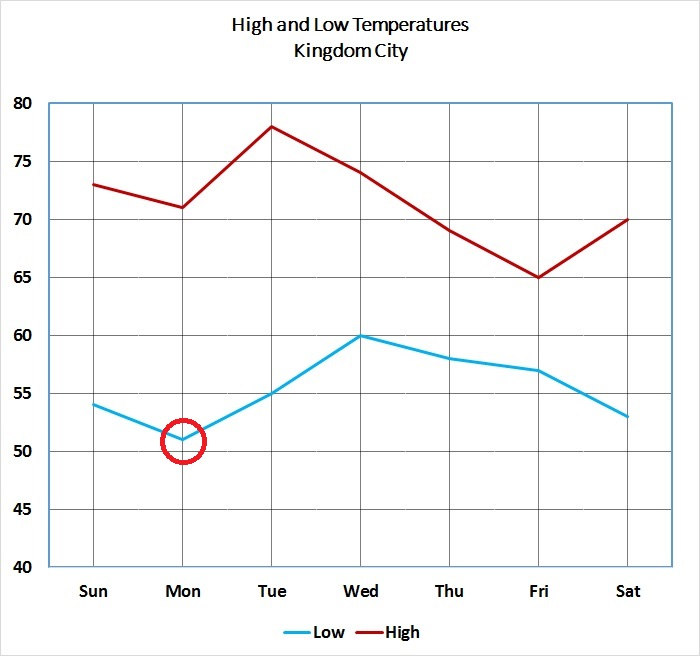To convert this to degrees Celsius, set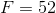and substitute in the given formula: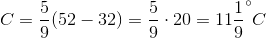degrees Celsius. The closest choice is.

### Example Question #6 : How To Read GraphsRefer to the above graph, which shows the high and low temperatures for Kingdom City over a one-week period. The temperatures at left are given in degrees Fahrenheit.

Temperatures given in degrees Fahrenheit can be converted to the Celsius scale using the formulaWhat was the difference, in degrees Celsius, between the highest temperature and the lowest temperature for the week (to the nearest whole degree)?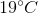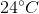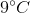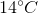Explanation:

As can be seen below, the highest temperature for the week was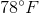, on Tuesday; the low temperature,, on Monday.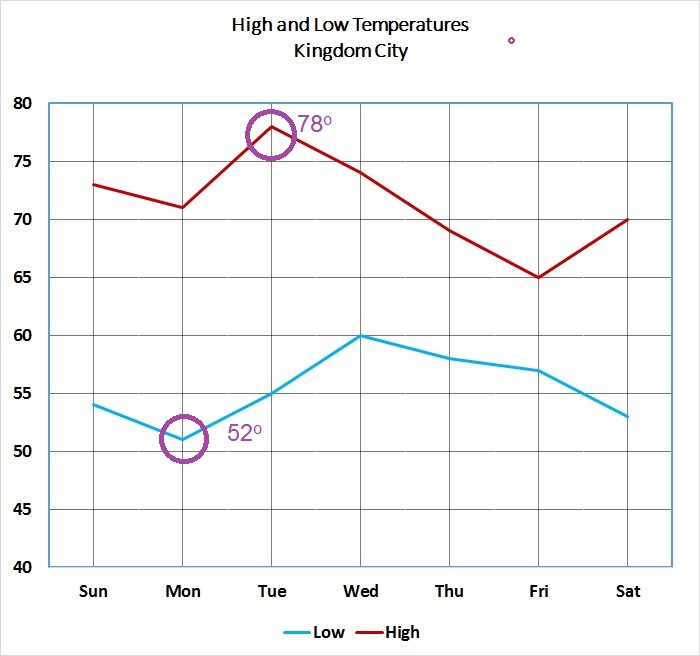To convert the former to degrees Celsius, set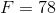and substitute in the given formula: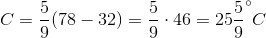.

To convert the latter, do the same, with..

The difference is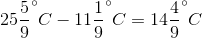, which rounds to.